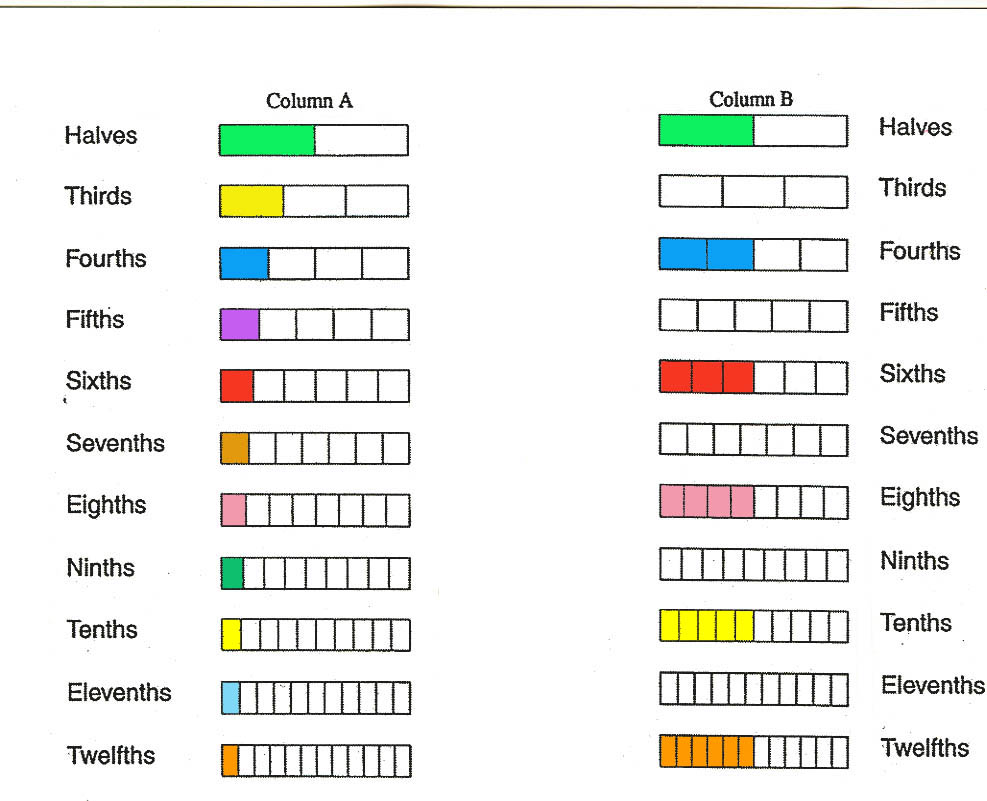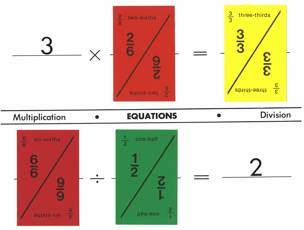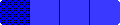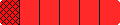Home Reproducible Materials and Activity Sheets Grades 5 and up

Below there is a link for the sheet of Blank Fraction Bars and links for six activities for shading Blank Fraction Bars to illustrate various relationships.

The following sheet of Blank Fraction Bars has been colored to show fraction relationships. The first column shows decreasing shaded amounts for fractions that get smaller and closer to zero. The second column shows bars that are half shaded and represent fractions equal to 1/2.The following file has Blank Fraction Bars for all the proper fractions with denominators from 2 through 12. This is designed to be used with the first four of the following six activities and also can be used for forming additional activity sheets.

Blank Fraction Bars

The following six files have activity sheets for the topics of equality, inequality, addition, subtraction, multiplication, and division of fractions.

EQUATIONS MATS

Use the Addition and Subtraction mat and one of the 10 Multiplication/Division mats to form equations. Select 10 arbitrary Fraction Bars or Fraction Bars Playing Cards and try to place them on the 10 blanks of the two mats to from equations (One example for two mats is shown below). If necessary, take one or more bars (cards) to form the 10 equations with as few as possible.TIC-TAC-TOE MATS

The object of Fraction Tic-Tac-Toe (or Mixed Number Tic-Tac-Toe) is to try to obtain three numbers side-by-side in a row column or diagonal on the mat.  As in any game of Tic-Tac-Toe the first person to do this wins the game. To play this game using either one of the two mats some method is needed to randomly select from the 144 numbers on the mat. One way to do this is to run off a copy of the mat and cut out the 144 numbers. Then place them in a sack or box for the players to randomly select one on each turn.

width="100%"width="100%"